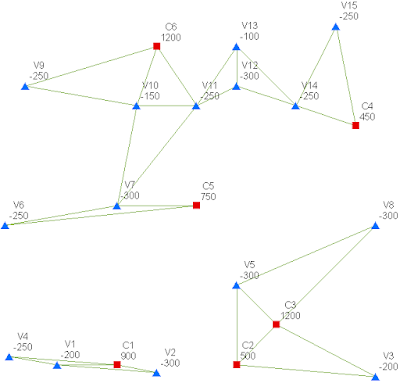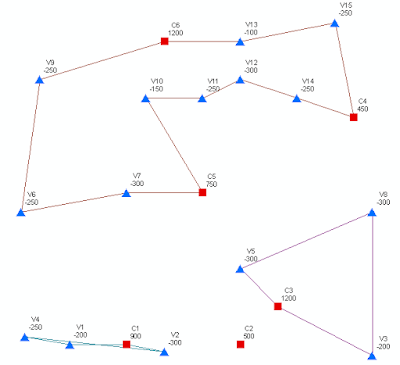## August 22, 2010

### Designing an Electrical Network

Earlier this year, the Fédération Française des Jeux Mathématiques organized a game called “Designing an Electricity Transportation Network.” The problem gives the locations in Euclidean coordinates of 6 power plants and 15 cities, along with the power production capabilities of the power plants and the power requirements of the cities. The goal of the problem is to find a network providing redundant power to all cities at minimum cost. The English version of the problem is here.

My attempt at solving the problem gave birth to the 'triangulator' algorithm based on the Traveling Salesman Problem (TSP). I observed that if all the points were connected with the TSP path, then the network was redundant in case of failure of one power line, and all the points were connected with the shortest possible path. Because more power is produced than is required, I wanted to divide the points into clusters to see if it was cheaper to have several power networks instead of one big network.

An exploratory step was to triangulate the surface defined by the set of all nodes using Delaunay's method. In this case, though it is not always true, the TSP is a subset of the Delaunay triangulation. My hunch was that all the smaller TSP solutions would be subsets of the triangulation as well.The Delaunay triangulation. Blue triangles are cities, and red squares are power stations. Numbers indicate supply (+) or demand (-) in megawatts.

The algorithm starts with a list of unpowered nodes and a list of power clusters. Initially all the cities are part of the unpowered nodes list, and the power plants are part of the power clusters list. A while loop runs until there are no more unpowered nodes. Within the loop, for each powered cluster s and for each point p in the cluster, the two nearest points i and j are found satisfying the following conditions:

• either i or j or both are unpowered
• i does not equal j
• the total power of the cluster that might be formed by s together with i and j is positive
• the perimeter of the triangle defined by p, i and j is the smallest possible perimeter among all current choices
Similarly to how minimum spanning trees are built at separate locations by Sollin’s algorithm, the triangulator is a greedy method that builds minimum-perimeter spanning triangulations. The triangles are chosen in such a way as to minimize the total sum of triangle perimeters. The algorithm terminates when there are no unpowered nodes left. It creates three separate self-sufficient clusters containing 12 triangles, which are not, however, a subset of the Delaunay triangulation.Output of triangulator showing the 12 triangles of minimum spanning triangulation.

The final step, performed by hand, was to find the TSP tour among the points in each cluster. Node C2 was left out because C3 provides enough power for that cluster already. The total length of this solution is 4,597.62 km, which is slightly larger than the un-clustered TSP solution of 4572.85 km.TSP tours of triangulator clusters

The winning solutions, which did not ignore the problem constraints like I did, are here:

Both solutions made use of additional points called Fermat points (aka Steiner, aka Torricelli). The Ramis-Straziota-Zaourar solution had an impressive linear programming setup and a very neat way to check network feasibility by modeling the network as a graph and using the Ford-Fulkerson algorithm to find augmenting paths.

Thanks to Matt Tang, my partner on this project, who helped program a brute-force attack on this problem, and to Alex Engau, our instructor in Network Flows at UC Denver (Spring 2010) for helpful advice and an awesome class.